﻿ Performing Analysis - MATLAB For Dummies, 2nd Edition

Chapter 20

# Performing Analysis

IN THIS CHAPTER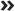Working with linear algebraUsing calculusResolving differential equations

Chapter 19 introduces you to the Symbolic Math Toolbox and shows you how to use it to perform a number of tasks. This chapter expands on some of the information presented in Chapter 19. In fact, before you proceed, make sure that you have the Symbolic Math Toolbox installed. The student version of MATLAB comes with the Symbolic Math Toolbox installed by default — otherwise, you must install it manually, using the instructions found in the first section of Chapter 19.

This chapter doesn’t take a detailed look at any one particular area of solving equations, but it does give you a good start on working with linear algebra, calculus, and differential equations. Being able to solve these equations quickly and correctly can make a huge difference in the success of your project. Some of the concepts in this chapter are a little advanced, so you see links for additional information about many of them. In addition, Chapter 5 provides you with a lot of details about working with matrices in MATLAB, so a review of that chapter is helpful.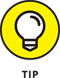You may think that these kinds of math are used only in high-tech environments, such as building a sub that can safely traverse the Marianas Trench. (You can read about James Cameron’s successful exploration of the trench using a custom submarine at https://news.nationalgeographic.com/news/2012/03/120325-james-cameron-mariana-trench-challenger-deepest-returns-science-sub/.) However, these equations are used in everyday life. For example, check out this story about how linear algebra can be employed to make a restaurant more profitable: https://smallbusiness.chron.com/restaurants-use-linear-programming-menu-planning-37132.html. Lest you think that this story is uncommon, check out this second story on calculating food costs at https://smallbusiness.chron.com/calculate-food-cost-restaurant-39551.html. The point is that you don’t really know when or where you’ll encounter these equations, so it’s a good idea to be prepared to use them. In many cases, the person employing the high-level math doesn’t even realize they’re doing it, as in 13 Examples Of Algebra In Everyday Life at https://studiousguy.com/examples-of-algebra-in-everyday-life/.

Using Linear Algebra

You use linear algebra to perform a number of tasks with matrixes in MATLAB. For example, you can determine whether a matrix is singular (a square matrix that doesn’t have a matrix inverse) or unimodular (a square integer matrix having determinant +1 or −1) by using the det() function. You can also reduce a matrix to determine whether it’s solvable — that it has an inverse (where matrix A * inv(A) results in an identity matrix; see Chapter 5 for additional matrix manipulation details). In fact, you can perform a relatively wide range of tasks using linear algebra with MATLAB and the Symbolic Math Toolbox; the following sections tell you how. (See Chapter 19 for details on the Symbolic Math Toolbox add-on.)

Working with determinants

Determinants are used in the analysis and solution of systems of linear equations. A nonzero value means that the matrix is nonsingular and that the system has a unique solution. A value of 1 usually indicates that the matrix is unimodular — that it’s a real square matrix, in other words. The function used to obtain the determinant value is det(). You supply a matrix, and the output value tells you about the ability to create a solution for that matrix.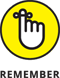Equally important is to know about the cond() function, which tests for singular matrices. Again, you supply a matrix as an input value, and the output provides a condition number that specifies the sensitivity of the matrix to error. An output value near 1 indicates a well-conditioned matrix. (The YouTube video at https://www.youtube.com/watch?v=JODxbi9B3tg provides an incredibly simplified illustration of the difference between a well-conditioned and an ill-conditioned matrix.)

To see how these two functions work together, type A = [1, 2, 3; 4, 5, 6; 7, 8, 9]; and press Enter to create a test matrix. This is a singular matrix. Type cond(A) and press Enter. The result of 1.1439e+17 (an approximation of infinity) tells you that this is a highly sensitive matrix — a singular matrix. Type det(A) and press Enter. The incredibly small output value of 6.6613e-16 (an approximation of zero) tells you that this is a singular matrix. (If you want to see a perfect singular matrix, try [0, 0, 0; 0, 0, 1; 0, 0, 0];. The cond() value is Inf, or infinity, and the det() value is 0.)

For comparison purposes, try a unimodal matrix. Type B = [2, 3, 2; 4, 2, 3; 9, 6, 7]; and press Enter to create the matrix. Type cond(B) and press Enter to see the condition number of 313.1721, which isn’t a perfect unimodal matrix, but it’s quite close. Type det(B) and press Enter to see the result of 1.0000, which is good (doesn’t approximate 0) for a unimodal matrix.

Performing reduction

Reduction lets you see the structure of what a matrix represents, as well as to write solutions to the system equations that the matrix represents. MATLAB provides the rref() function to produce the Reduced Row Echelon Form (RREF). (You can find out more about RREF at https://www.educba.com/matlab-rref/.) You can find an interesting tool to see the steps required to produce RREF using any matrix as input at https://www.emathhelp.net/calculators/linear-algebra/reduced-row-echelon-form-rref-caclulator/. The point is that you can perform reduction using MATLAB, and doing so requires only a couple of steps.

The first step is to create the matrix. In this case, the example uses a magic square. Type A = magic(5) and press Enter. The magic() function will produce a magic square of any size for you. (You can read about magic squares at https://mathworld.wolfram.com/MagicSquare.html). The output you see is

A =

17 24 1 8 15

23 5 7 14 16

4 6 13 20 22

10 12 19 21 3

11 18 25 2 9

The second step is to perform the reduction. Type rref(A) and press Enter. Any nonsingular matrix will reduce to identity, as follows:

ans =

1 0 0 0 0

0 1 0 0 0

0 0 1 0 0

0 0 0 1 0

0 0 0 0 1

You can use rref() to solve linear equations. In this case, if A*x=y and y=[1;0;0;0;0], then B=rref([A,y]) solves the equation. The following steps demonstrate how this works:

1. Type y=[1;0;0;0;0]; and press Enter.

2. Type A=magic(5); (in case you didn’t type it before) and press Enter.

3. Type B=rref([A,y]) and press Enter.

You see the following output:

B =

1.0000 0 0 0 0 -0.0049

0 1.0000 0 0 0 0.0431

0 0 1.0000 0 0 -0.0303

0 0 0 1.0000 0 0.0047

0 0 0 0 1.0000 0.0028

4. Type x=B(:,6) and press Enter.

You see the following output:

x =

-0.0049

0.0431

-0.0303

0.0047

0.0028

At this point, you want to test the equation.

5. Type A*x and press Enter.

You see the following output:

ans =

0.9999

-0.0001

-0.0001

-0.0001

-0.0001

Notice that the output values match the original value of y to within a small amount. In other words, the steps have proven the original equation, A*x=y, true.

Using eigenvalues

An eigenvalue (v) is an eigenvector of a square matrix. You see this form of math used in transformations as described at https://www.mathsisfun.com/algebra/eigenvalue.html. The variable A is a nonzero matrix. When v is multiplied by A, it yields a constant multiple of v that is commonly denoted by λ. Eigenvalues are defined by the following equation:

Av = λv

Eigenvalues are used in all sorts of ways, such as for graphics manipulation (sheer mapping) and analytic geometry (to display an arrow in three-dimensional space). You can read more about eigenvalues at https://mathworld.wolfram.com/Eigenvalue.html and https://en.wikipedia.org/wiki/Eigenvalues_and_eigenvectors.

To see how this works, you first need to create a matrix. Type A = gallery('riemann', 4) and press Enter. The gallery() function produces test matrices of specific sizes filled with specific information so that you can repeat test results as needed. The output of gallery() depends on the matrix size and the function used to create the matrix. (You can read more about gallery() at https://www.mathworks.com/help/matlab/ref/gallery.html.) The output from this particular call is

1 -1 1 -1

-1 2 -1 -1

-1 -1 3 -1

-1 -1 -1 4Obtaining the eigenvalue comes next. The output will contain one value for each row of the matrix. Type lambda = eig(A) and press Enter to see the eigenvalue of the test matrix, A, as shown here:

lambda =

-0.1249

2.0000

3.3633

4.7616

Understanding factorization

Factorization is the decomposition of an object, such as a number or polynomial. The idea behind factorization is to reduce the complexity of the object so that it’s easier to understand and solve. In addition, it helps you determine how the object is put together, such as its use for prime factorization (see https://www.calculatorsoup.com/calculators/math/prime-factors.php). You can read more about factorization at https://mathworld.wolfram.com/Factorization.html.

You perform factorization in MATLAB using the factor() function. You can use the factor() function in a number of ways: working with numbers and working with polynomials.

When working with a number, you simply provide the number as input to obtain a list of prime number values. For example, type factor(2) and press Enter. The output is 2 because 2 is a prime number. Type factor(12) and press Enter. The output is

ans =

2 2 3

because 2 * 2 * 3 equals 12. You can also handle the number symbolically. Type factor(sym(12)) and press Enter to see an output of

ans =

[2, 2, 3]When working with negative numbers, you use the symbolic form or you receive an error message saying the following:

N must be a real nonnegative integer.

Instead of entering the number directly, you type factor(sym(-12)) and press Enter to see an output of

ans =

[-1, 2, 2, 3]

You can use factor() on fractional values. Type factor(sym(14/3)) and press Enter to see an output of [2, 7, 1/3] because 2 * 7 * 1/3 would equal 14/3.

Polynomials require that you declare symbolic objects first by using syms. Type syms x y and press Enter to create the required objects. Type factor(x^2 + 2*x*y + y^2) and press Enter. The output is [x + y, x + y].

It’s possible to achieve particular results when using a factor mode with factor(). The following list shows what happens when you add the 'FactorMode' argument and a variable to the factor() call.

 Type To Get factor(3*x^2 - 2*x + 22) 3*x^2 - 2*x + 22 factor(3*x^2 - 2*x + 22, x, 'FactorMode', 'real') [3.0, x^2 - 0.66666666666666666666666666666667*x + 7.3333333333333333333333333333333] factor(3*x^2 - 2*x + 22, x, 'FactorMode', 'complex') [3.0, x - 0.33333333333333333333333333333333 + 2.6874192494328498841222044101013i, x - 0.33333333333333333333333333333333 - 2.6874192494328498841222044101013i] factor(3*x^2 - 2*x + 22, x, 'FactorMode', 'full') [3, x + (65^(1/2)*1i)/3 - 1/3, x - (65^(1/2)*1i)/3 - 1/3]

You can combine the output of the 'full' mode with vpa() to approximate a result. For example, using the 'full' mode results of 3*x^2 - 2*x + 22 with vpa(), you obtain

[3.0, …

x - 0.33333333333333333333333333333333 + …

2.6874192494328498841222044101013i, …

x - 0.33333333333333333333333333333333 - …

2.6874192494328498841222044101013i]

as a result.

Employing Calculus

Calculus can solve myriad problems that algebra can't. It’s really the study of how things change. This branch of math is essentially split into two pieces: differential calculus, which considers rates of change and slopes of curves; and integral calculus, which considers the accumulation of quantities and the areas between and under curves. The following sections show how you can use MATLAB with the Symbolic Math Toolbox to solve a number of relatively simple calculus problems.

Working with differential calculus

MATLAB offers good differential calculus support. The example in this section starts with something simple: univariate differentiation. (Remember that univariate differentiation has a single variable.) MATLAB supports a number of forms of differential calculus — each of which requires its own set of functions. In this case, you use the diff() function to perform the required tasks. The following steps help you perform a simple calculation:

1. Type syms x and press Enter.

MATLAB creates a symbolic object to use in the calculation.

2. Type f(x) = sin(x^3) and press Enter.

Doing so creates the symbolic function used to perform the calculation. Here’s the output you see:

f(x) =

sin(x^3)

3. Type Result = diff(f) and press Enter.

The output shows the result of the differentiation:

Result(x) =

3*x^2*cos(x^3)Result(x) is actually a symbolic function. You can use it to create a picture of the output.

4. Type plot(Result(1:50)) and press Enter.

Figure 20-1 shows the plot created from the differentiation of the original symbolic function.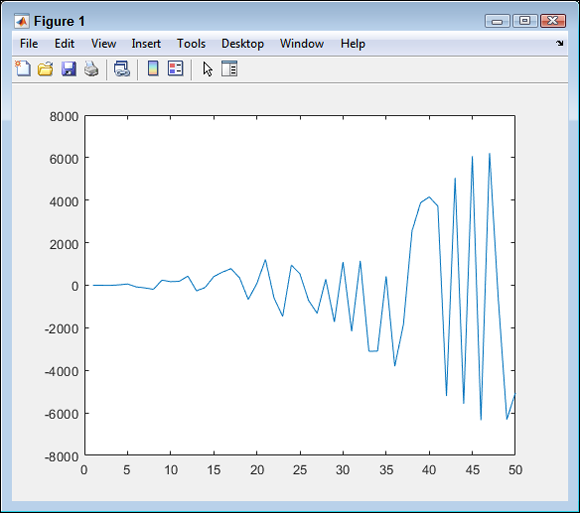FIGURE 20-1: The plot shows the result of the differentiation.

Using integral calculus

You’ll also find great integral calculus support in MATLAB. As does the example in the preceding section, the example in this section focuses on a univariate calculation. In this case, the example relies on the int() function to perform the required work. The following steps help you perform a simple calculation:

1. Type syms x and press Enter.

MATLAB creates a symbolic object to use in the calculation.

2. Type f(x) = (x^3 + 3*x^2) / x^3 and press Enter.

The symbolic function that you create produces the following output:

f(x) =

(x^3 + 3*x^2)/x^3

3. Type Result = int(f, x) and press Enter.

Notice that you must provide a symbolic variable as the second input. The output shows the following symbolic function as the result of the integration:

Result(x) =

x + 3*log(x)

4. Type plot(Result(1:50)) and press Enter.

Figure 20-2 shows the plot created from the integration of the original symbolic function.

Working with multivariate calculus

The “Working with differential calculus” section, earlier in the chapter, shows how to work with a single variable. Of course, many (if not most) problems don’t involve just one variable. With this idea in mind, the following steps demonstrate a problem with more than one variable — a multivariate example:

1. Type syms x y and press Enter.

MATLAB creates the two symbolic objects used for this calculation.

2. Type f(x, y) = x^2 * sin(y) and press Enter.

This symbolic function accepts two inputs, x and y, and uses them to perform a calculation. Here’s the output from this step:

f(x, y) =

x^2*sin(y)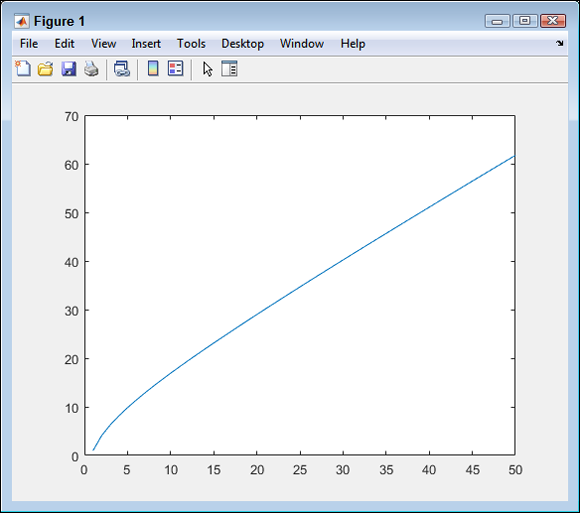FIGURE 20-2: The output is usually a curve.

3. Type Result = diff(f) and press Enter.

The output shows the result of the differentiation:

Result(x, y) =

2*x*sin(y)

In this case, Result(x, y) accepts two inputs, x and y. As before, you can create a picture from the output of Result().The example shows the derivative with respect to x, which is the default. To obtain the derivative with respect to y (df/dy), you type diff(f, y) instead.

4. Type plot(Result(1:50, 1:50)) and press Enter.

Figure 20-3 shows the output of the plot created in this case.Notice that in this case, you must provide both x and y inputs, which isn’t surprising. However, the two vectors must have the same number of elements or MATLAB will raise an exception.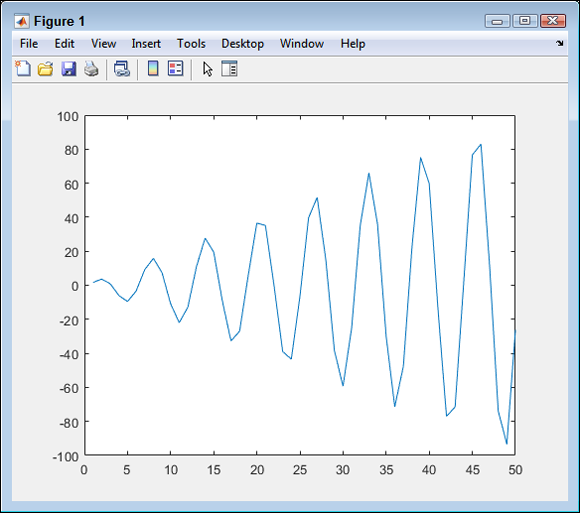FIGURE 20-3: Using two variables requires vectors of equal size.

Solving Differential Equations

When working with differential equations, MATLAB provides two different approaches: numerical and symbolic. The following sections demonstrate both approaches to solving differential equations. Note that these sections provide just an overview of the techniques; MATLAB provides a rich set of functions to work with differential equations.

Using the numerical approach

When working with differential equations, you must create a function that defines the differential equation. This function is passed to MATLAB as part of the process of obtaining the result. There are a number of functions you can use to perform this task; each has a different method of creating the output. You can see a list of these functions at https://www.mathworks.com/help/matlab/ordinary-differential-equations.html and additional information at https://www.mathworks.com/help/matlab/numerical-integration-and-differential-equations.html. The example in this section uses ode23(), but the technique works for the other functions as well (see https://www.mathworks.com/help/matlab/math/choose-an-ode-solver.html for a list of standard functions).MATLAB has a specific way of looking at your function. The order in which the variables appear is essential, so you must make sure that your function is created with this need in mind. The example in this section simplifies things to avoid the complexity of many examples online and let you see the process used to perform the calculation. The following steps get you started:

1. Type Func = @(T, Y) cos(T*Y) and press Enter.

You see an output of

Func =

function_handle with value:

@(T,Y)cos(T*Y)

The requirements for the differential function are that you must provide an input for time and another input containing the values for your equation. The time value, T, is often unused, but you can use it if you want. The variables can consist of anything required to obtain the result you want. In this case, you input a simple numeric value, Y, but inputs can be vectors, matrices, or other objects as well.

2. Type [TPrime, YPrime] = ode23(Func, [-10, 10], .2); and press Enter.

When using ode23(), you must provide a function — Func in this case — as input. As an alternative, you provide the name of the file containing the function. The second argument is a vector that contains the starting and ending times of the calculation. The third argument is the starting input value for the calculation.

The TPrime output is always a vector that contains the time periods used for the calculation. The YPrime output is a vector or matrix that contains the output value or values for each time period. In this case, YPrime is a vector because there is only one output value.

3. Type plot(TPrime, YPrime) and press Enter.

You see the plotted result for this example, as shown in Figure 20-4.

Using the symbolic approach

When working with the symbolic approach, you rely on the functionality of the Symbolic Math Toolbox to speed the solution and make it easier to solve. Even though the solution in the previous section looks easy, it can become quite complicated when you start working with larger problems. The symbolic approach is straightforward because you use dsolve() (see https://www.mathworks.com/help/symbolic/dsolve.html). The following steps show a simple example of using dsolve() to create a differential solution and then plot it (this technique will generate a warning message that you can ignore; it’s the easiest way to perform this task):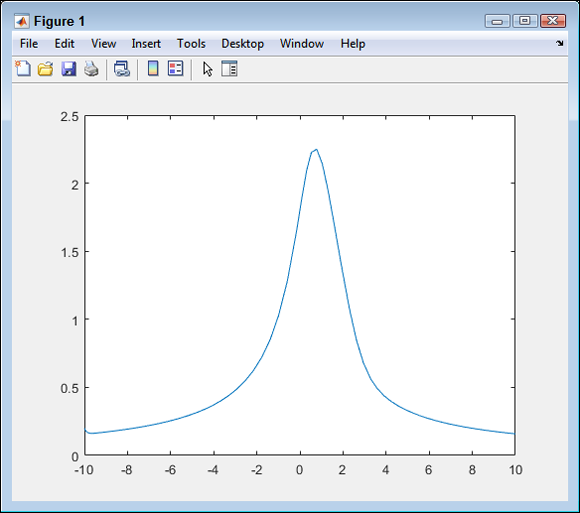FIGURE 20-4: Obtaining a result using the numeric approach.

1. Type Solution = dsolve('Dy=(t^2*y)/y', 'y(2)=1', 't') and press Enter.

The arguments to dsolve() consist of the equation you want to solve, the starting point for y (a condition), and the name of the independent variable. You see the following output from this entry:

Solution =

t^3/3 - 5/3

2. Type Values = subs(Solution, 't', -10:.1:10); and press Enter.

Solution simply contains the solution to the equation given the conditions you provide. The subs() function substitutes values for t one at a time. In this case, the values range from –10 to 10 in 0.1 increments. When this command completes, Values contains a list of results for the values you provided that you can use as plot points.

3. Type plot(Values) and press Enter.

You see the output shown in Figure 20-5.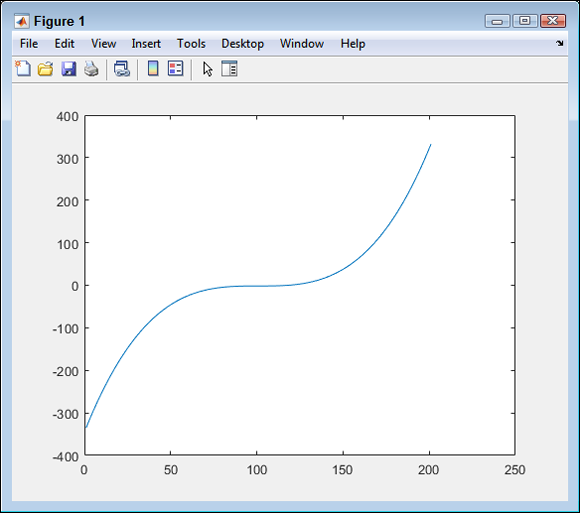FIGURE 20-5: Obtaining a result using the symbolic approach.